## Example Questions

### Example Question #1 : How To Find Amount Of Profit

Mary buys a car from a mean salesman who charges her 12% over the original price of a $15,000 car. Luke buys the same car from a much nicer salesman who gives him an 8% discount off of the original price. How much more does Mary spend on the car than Luke does? Possible Answers:$3000

$2000$2500

$1200 Correct answer:$3000

Explanation:

12% of 15,000 is 0.12 * 15,000 = 1800.

8% of 15,000 is 0.08 * 15,000 = 1200; therefore in total, Mary spent 1800 + 1200 = $3000 more. ### Example Question #1 : How To Find Amount Of Profit A grade school pays Mr. Day a salary of$24,585 per school year.  Each school year contains 165 days.  Suppose Mr. Day is sick for a week (5 work days) and the school doesn't have to pay him for those days.  Instead, they must pay a substitute teacher to teach his classes.  They pay the substitute $90 per day. Totally, how much does the school save for the week Mr. Day is sick? Possible Answers:$295

The school must pay $59 extra for the substitute teacher.$59

$205 The answer cannot be determined from the given information. Correct answer:$295

Explanation:

Divide Mr. Day's salary by 165 to determine how much the school pays him per day: Mr. Day makes $149 per day. They only have to pay substitute$90 per day, saving them $59 per day. To figure out how my they save totally, multiply by 5 to get how much they save for the week Mr. Day is sick:$295.

### Example Question #1 : How To Find Amount Of Profit

What percentage of profit is made on a product sold for $20 if its overall production cost was$17.50?

25%

87.5%

15.252%

14.29%

46.67%

14.29%

Explanation:

To find the profit percentage, you must first determine the amount of profit made on this transaction.  If the sale price was $20 and the production cost$17.50, then the profit made was: 20 -17.5 = $2.50. The profit percentage is determined by dividing the amount of profit made by the original price, or 2.5 / 17.5 = (approx.) 0.14286 or 14.29%. ### Example Question #4 : Profit Margin Manufacturer X has a base monthly operating cost of$30,000.  It makes only one product, which costs $5 per piece in addition to the base operational costs for the plant. These products are each sold for$15 apiece.  How many products must the company sell in order to break even in any given month?

3,000

10,000

2,500

1,500

2,000

3,000

Explanation:

Begin by translating your problem into an equation.  What we want to ascertain is the situation when the profit equals exactly 0.  The profit and loss for a given month can be summarized by the following equation:

(Total product revenue) - (Base operating costs) - (Individual costs for products) = 0

Given our data, we can rewrite this:

15 * x - 30,000 - 5 * x = 0

Combine like terms:

10 * x - 30,000 = 0

Isolate x:

10 * x = 30,000; x = 3,000.

Therefore, the manufacturer must sell at least 3,000 items per month in order to "break even."

### Example Question #1 : Profit Margin

A new t-shirt has a total cost of 8 dollars for a given retailer.  Its current price is $15. If the retailer discounts the cost of the shirt by 20%, how many must it sell in order to make the same amount of profit as when it sold 300 of the shirts at the original price? Possible Answers: 525 360 395 400 None of the other answers Correct answer: 525 Explanation: First, we must ascertain the original profit. Per shirt, the retailer made 15 – 8, or$7.  Selling 300 shirts, it made a profit of $2,100. The new price, discounted by 20% is equal to 80% of the original price, or 15 * 0.8 =$12.  This yields a profit of $4 per shirt. To ascertain the number of sales needed to make$2,100 in profit, we must solve the following equation:

4x = 2100; x = 525 shirts

### Example Question #1 : How To Find Amount Of Profit

A laptop computer costs $235 to manufacture. If it is sold for$578, what is the percent of profit made on the item?

145.96%

53.38%

59.34%

245.96%

343%

145.96%

Explanation:

The amount of profit made on the item is 578 – 235 = $343. This is 343/235 or (approximately) 1.4596, which is 145.96% of the original price. ### Example Question #2 : Profit Margin Sally buys a dress that is a 20% discount from the original price. If she sells it at a 10% markup from her purchase price and profits$10 from the sale, what was the original price of the dress?

125

110

100

120

20

125

Explanation:

Set Original = O

and Discount = D

then

D = (1 – 20%) x O = 0.80 x O

and Profit = $10 so: 10 = ((1 + 10%) x D) – D = 1.1 * D – D = 0.1D D = 100 and O = D / 0.8 = 125 ### Example Question #51 : Percentage A shirt costs$12 to manufacture. If the marketing and sales costs are a 75% addition to this manufacturing cost. What is the minimum price necessary for making a 50% profit?

$31.5$21

$10.5$18

$16.5 Correct answer:$31.5

Explanation:

Based on the prompt, we know that the additional marketing and sales costs are 12 * 0.75 = 9; therefore, the total cost for purchase and sale is 12 + 9 = $21. To make a 50% profit, we must make 21 * 0.5, or$10.5, on the sale; thus the shirt must be sold for 21 + 10.5, or $31.5. ### Example Question #1 : How To Find Amount Of Profit A factory has fixed costs of$25,000 per month. It manufactures widgets at a total manufacturing cost of $45 per widget. They are sold at$60. How many widgets must be sold in any given month in order to break even?

1667

1666

555

556

1753

1667

Explanation:

Let's first represent the total costs per month:

C = 25000 + 45n, where n is the number of widgets manufactured.

The profit can be represented as 60n – C or 60n – 25000 – 45n = 15n – 25000. Now, we merely have to solve this for 0 in order to find the "break even" line.

15n – 25000 = 0 → 15n = 25000 → n = 1666.67.  We must sell a whole number of widgets, so it must be 1667.

### Example Question #1 : Profit Margin

A boy with a lemonade stand sells cups of lemonade for a quarter each. He has bought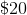worth of supplies and is able to make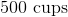of lemonade with the supplies. If he has to pay a business tax of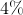for each cup he sells, how many cups will he have to sell in order to break even?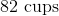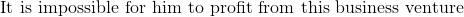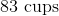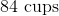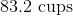To solve this problem we must first find out how cups he must sell without tax to break even. If each cup of lemonade costs a quarter and he has spent $20 on supplies, that means in order to make back the original$20 he spent, he must sell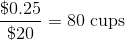.  Due to this 4% business tax, he must sell 4% more in order to break even. To find that amount, we simply multiply that 4% by the number of cups he must sell without tax to break even,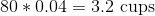. He must sell an additional 3.2 cups in order to break even, however it is impossible to sell 0.2 of a cup of lemonade, therefore he must sell a minimum of 84 cups of lemonade in order to break even.